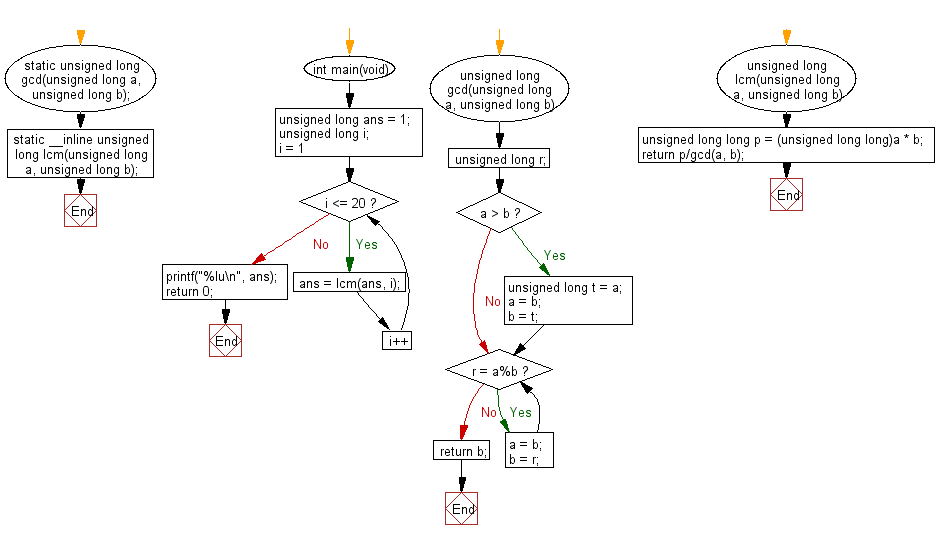﻿ C Program: Smallest positive number divisible by 1-20# C Exercises: Find the largest palindrome made from the product of two 3-digit numbers

## C Programming Practice: Exercise-21 with Solution

2520 is the smallest number that can be divided by each of the numbers from 1 to 10 without any remainder.
Write a C programming to find the smallest positive number that is evenly divisible by all of the numbers from 1 to 20?.

C Code:

``````/* Copyright (c) 2009, eagletmt, Released under the MIT License <http://opensource.org/licenses/mit-license.php> */
#include <stdio.h>
static unsigned long gcd(unsigned long a, unsigned long b);
static __inline unsigned long lcm(unsigned long a, unsigned long b);
int main(void)
{
unsigned long ans = 1;
unsigned long i;
for (i = 1; i <= 20; i++) {
ans = lcm(ans, i);
}
printf("%lu\n", ans);
return 0;
}
unsigned long gcd(unsigned long a, unsigned long b)
{
unsigned long r;
if (a > b) {
unsigned long t = a;
a = b;
b = t;
}
while (r = a%b) {
a = b;
b = r;
}
return b;
}
unsigned long lcm(unsigned long a, unsigned long b)
{
unsigned long long p = (unsigned long long)a * b;
return p/gcd(a, b);
}
``````

Sample Output:

```232792560
```

Flowchart:## C Programming Code Editor:

What is the difficulty level of this exercise?

Test your Programming skills with w3resource's quiz.

﻿

## C Programming: Tips of the Day

Maximum value of int:

In C:

```#include <limits.h>
then use
int imin = INT_MIN; // minimum value
int imax = INT_MAX;```

or

```#include <float.h>

float fmin = FLT_MIN;  // minimum positive value
double dmin = DBL_MIN; // minimum positive value

float fmax = FLT_MAX;
double dmax = DBL_MAX;```

Ref : https://bit.ly/3fi8yk9